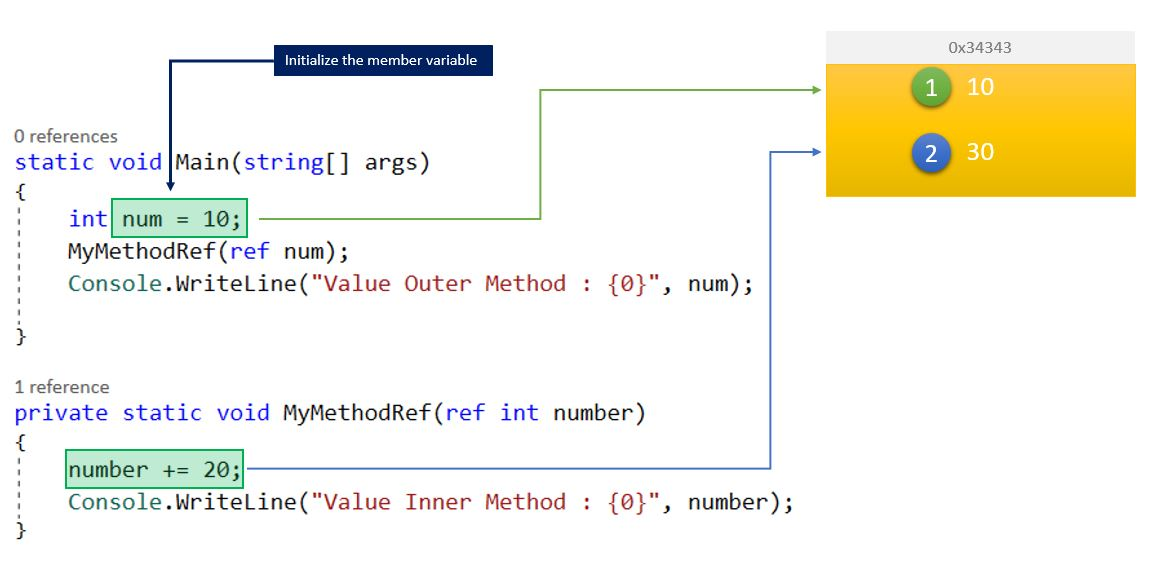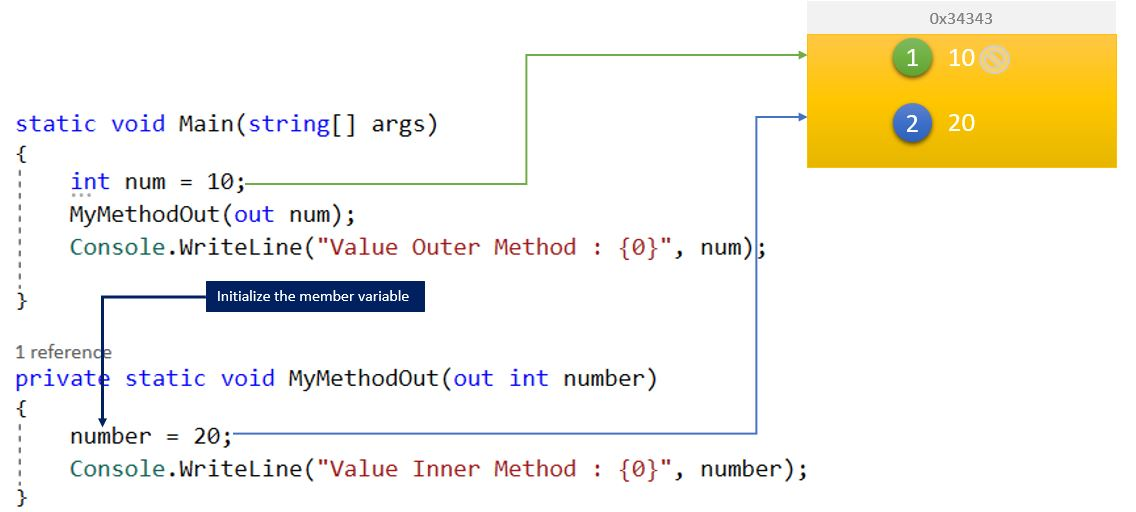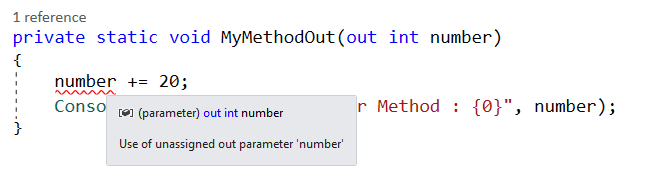# Back to Basics – What is the difference between Ref and Out Keyword in C#?

Continuing with the Back to Basics series, here is another common and frequently asked question. What is the difference between Ref & Out C#? or When should we use ref over out? We used it often, and we know both ref and out parameters are used to pass arguments within methods. Though it’s a very common question, I still find confusion while answering this question. In this post, we will try to understand the difference in a simpler way.

First of all, keep in mind, Both out and ref are used to returning values and both of them passes variables by reference only. Also, they worked almost in a very similar way. So, don’t get confused in this part at first place.

Let’s get into the details by starting with Ref Keyword.

While we pass the parameters using ref keyword, a reference to the variable is passed. Thereafter, any modification to the variable values will affect across. It means the parameter references the same memory location as the original variable irrespective of the methods.

Consider this following example.

```static void Main(string[] args)
{
int num = 10;
MyMethodRef(ref num);
Console.WriteLine("Value Outer Method : {0}", num);

}

private static void MyMethodRef(ref int number)
{
number += 20;
Console.WriteLine("Value Inner Method : {0}", number);
}
```

MyMethodRef() takes the number as ref parameter, which is taking ref num as an argument. Therefore, it is using the same reference point the num variable, which mean, any changes to the number will reflect across. With that, the below output is very clear and understandable.In this case, as both numbers are sharing same reference point; the value will remain same and updated.

Things that you need to consider while using Ref is,

1. You must have to initialize the parameter or argument first before you pass it as a reference.
2. It’s a two-way communication, the parameter passing through contains the values passed by the method and any modification passes back to the main method – that is because of simply having a reference.

On the other hand, out parameter works in a similar way, however, you need to initialize the variable inside the method which is called, and once the reference is passed, the original value (if there is any) will be ignored, and reference will have updated value from the method which is called.

If we execute the following code

```static void Main(string[] args)
{
int num = 10;
MyMethodOut(out num);
Console.WriteLine("Value Outer Method : {0}", num);

}

private static void MyMethodOut(out int number)
{
number = 20;
Console.WriteLine("Value Inner Method : {0}", number);
}
```

We will have output like belowMyMethodout() takes the number as ref parameter, which is taking ref num as an argument. Therefore, it is using the same reference point the num variable, however, as it is reinitialized, the passed value is ignored. With that, only updated values reflect the reference.In case you want perform any operation directly to the parameter, you will get following error.To Summarize, Ref required initialization of member variable before you are passing it as the reference, whereas for out, it is not mandatory. You need to initialize it in the called method. When you are using ref, referenced variables values is maintained across all method, whereas for Out method, Called by methods value is ignored.

Hope this helps!

DON'T MISS OUT!

Best Tips & Tricks on Microsoft Technology Platform